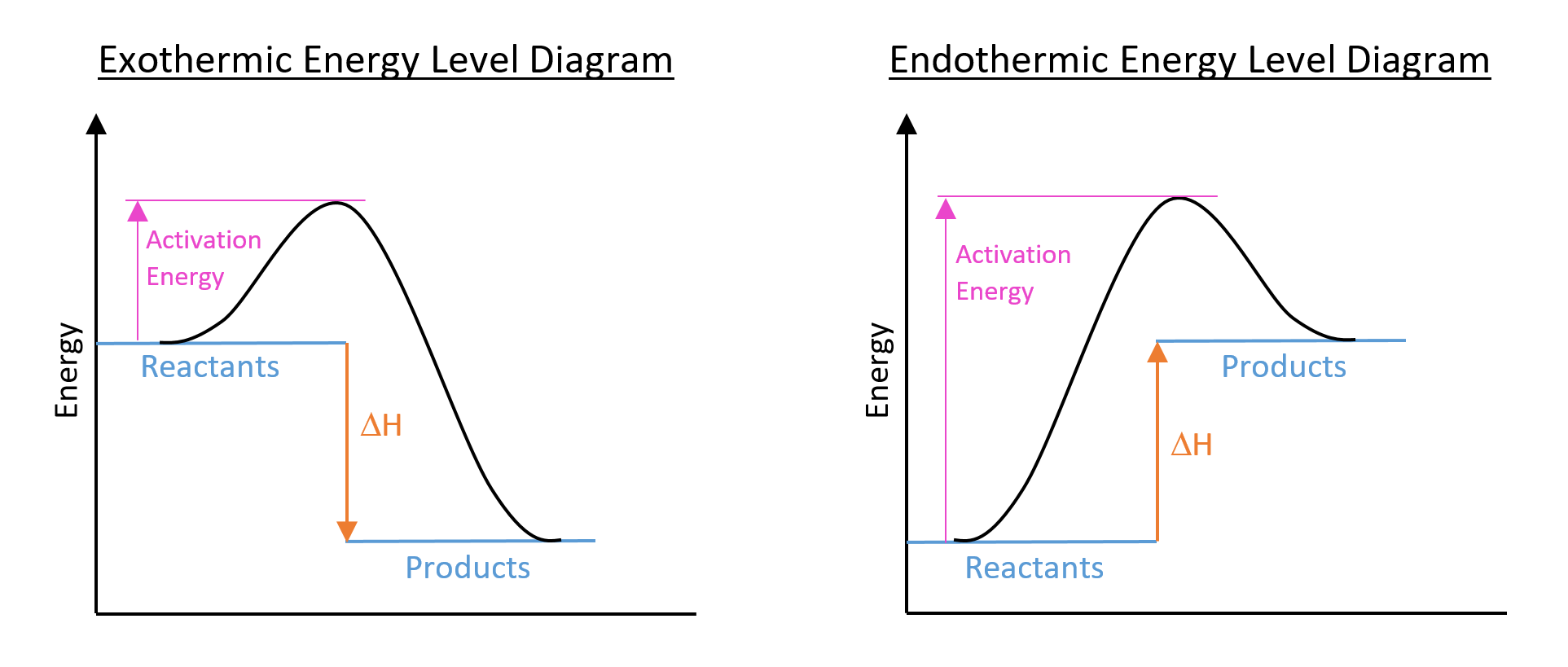Top ↑#### CONTENTS# Thermochemistry:

## EXOTHERMIC AND ENDOTHERMIC REACTIONS

Exothermic Reactions:

DEFINITION: Exothermic Reaction

An exothermic reaction is one which gives out energy to the surroundings, usually in the form of heat and usually shown by a rise in temperature.

View Glossary

Examples of Exothermic Reactions:

• Combustion
• Neutralisation reactions
• Hydration of anhydrous copper sulphate

Endothermic Reactions:

DEFINITION: Endothermic Reaction

An endothermic reaction is one which takes in energy from the surroundings, usually in the form of heat and usually shown by a fall in temperature.

View Glossary

Examples of Endothermic Reactions:

• Photosynthesis
• Dissolving certain salts in water (e.g. potassium chloride, ammonium nitrate)
• Thermal decomposition (e.g. converting calcium carbonate into calcium oxide (quicklime))

## ENTHALPY CHANGE

ΔH (read as 'delta H') is the symbol for the enthalpy change of a reaction. Enthalpy change refers to the change in energy in a reaction. ΔH is negative for an exothermic reaction (i.e. the products have less energy than the reactants) and positive for an endothermic reaction (i.e. the product have more energy than the reactants).

• ΔH is negative for exothermic reactions
• The difference in energy is given out as heat.
• Therefore, the temperature rises.
• ΔH is positive for endothermic reactions
• The extra energy needed to form the products is taken in from the surroundings.
• Therefore, the temperature falls.

Energy Level Diagrams:

Enthalpy change can be illustrated using an energy level diagram:Sometimes, a simplified version of the above diagrams are drawn, without the reaction pathway curve and activation energy drawn on.

DEFINITION: Activation Energy

Activation energy is the minimum energy required for reactants to undergo a chemical reaction. It can be lowered by using a catalyst.

View Glossary

## CALORIMETRY CALCULATIONS

Formula for Energy Change:

FORMULA:

q = mcDeltaT

Where:
q = energy change
m = mass of water
c = specific heat capacity
DeltaT = temperature change

Specific Heat Capacity (c) is the quantity of energy, in Joules (J), needed to change the temperature of one gram (g) of a substance by one degree Celsius (oC).

e.g. The specific heat capacity of water is 4.18 J/g/oC

Formula for Enthalpy Change:

FORMULA:

DeltaH = -q/n

Where:
DeltaH = enthalpy change
q = energy change
n = number of moles

## BOND ENERGY

We can work out the energy needed to break different chemical bonds. This is called the bond energy. These bond energies can be used to calculate ΔH for a reaction.

DEFINITION: Bond Energy

Bond energy is the amount of energy required to break one mole of a stated bond, measured in kJ/mol. It can be used to calculate ΔH for a reaction.

View Glossary

Bond energies are average values for the bonds in different molecules. Therefore, these calculations only give us a rough value for ΔH.

Making bonds is EXOTHERMIC
Breaking bonds is ENDOTHERMIC

Back to Chemistry Home